Size Estimation (Selectivity) in DBMS

We use different types of operations in a query. Depending upon the type of joins and operators, the size of the result set varies. Let us see them below.

Selection

Suppose we have equality selection operation, σ A=value (T). Now the size estimation of result is as simple as explained above. Suppose A is a key column. Then size estimation equals to one. This is because each record in a table has distinct value for column A.
Suppose we have comparative operator in selection operation, σ A<=value (T). Suppose max and min value of column A is also stored in data dictionary. Then size estimate is calculated as

Suppose we have comparative operator in selection operation, σ A<=value (T). Suppose max and min value of column A is also stored in data dictionary. Then size estimate is calculated as

n r *(value – min (A)/(max(A)  - min(A)), if value> min(A)
0, if value<min (A)

For example, suppose column age has max value 25 and min value 18. We have selection condition, age<= 20. Total number of records in table is 100. Then size estimate is calculated as,

100 * (20 – 18) / (25-18) ≈ 29

i.e.; approximately 29 records can be fetched from the table with age <=20. Suppose the condition is age<15, then the probability of getting such records is zero; no records are present with such age.

Suppose we have theta join on table T. Then the selectivity or probability of selecting the records with join condition is given as SA / nr. Suppose we have multiple theta joins with AND condition, conjunction. Then the selectivity is given as nr *(S1 * S2*… S n)/n nr.

Architecture of Database

Suppose we have EMP table with 100 records and we have theta join on AGE, CLASS_ID. Suppose selectivity of AGE is 5 and CLASS_ID is 10. Then selectivity of conjunctive join condition is given as 100 * (5*10)/100100 which is very small.

Similarly, in the case of disjunction (OR condition), it is given as nr *(1 – (1-S1/ nr)*(1-S2/ nr)… (1-Sn/nr).
In the case of negation (NOT condition), it is nr – size (σ θ (T))

Join

Suppose we have join operation on two tables T and S. Then we have different cases for estimating the selectivity of records

• Cartesian Product : The Cartesian product will have records copied from both tables and hence it is nt*ns records. It copies ST + SS bytes from each records. For example, suppose we have 100 records in EMP and 5 records in DEPT. Say selectivity of table EMP is 5 (columns) and DEPT is 3 (columns). Then cartesian product will have 500 records into the result set, copying 8 bytes of each record.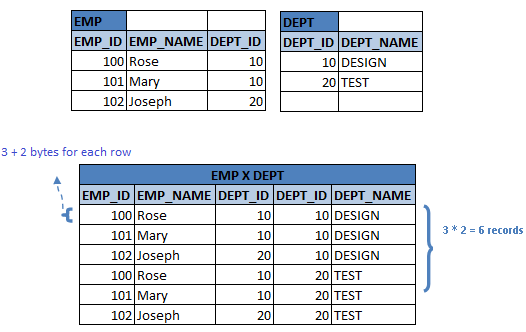• T∩S = ?, then if we have join on T and S, T ∞ S, it will be same as Cartesian product. i.e.; each record in T and S are distinct and hence their join will have distinct values.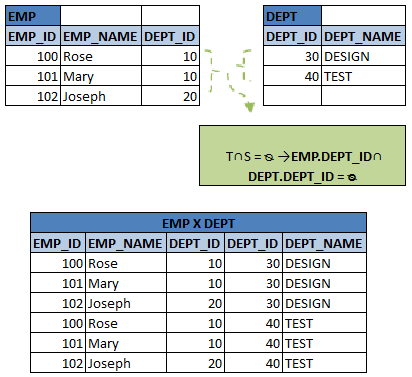• T∩S = key (T), then each record in S will be joined with T, and hence the number of records in T ∞ S will be utmost equal to number of records in S. For example number of records in EMP = 5 and EMP EMP_ID ∩ EMP MGR_ID = key of EMP = EMP_ID. Then the join between EMP ∞ EMP_ID = MGR_ID   EMP will be same as number of managers in EMP (i.e.; S)
Database Management System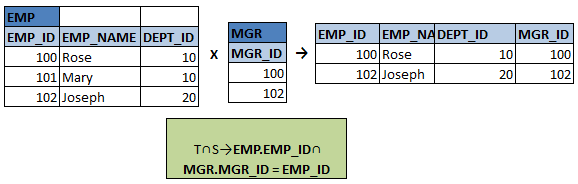• T∩S =Foreign key in T, then each record in S will be joined with T, and hence the number of records in T ∞ S will be equal to number of records in S. For example number of records in EMP = 5 and DEPT = 3.  Then the DEPT_ID of DEPT is the foreign key in EMP. Hence the join between EMP ∞ DEPT_ID = DEPT_ID DEPT will be same as number of employees in EMP (i.e.; T)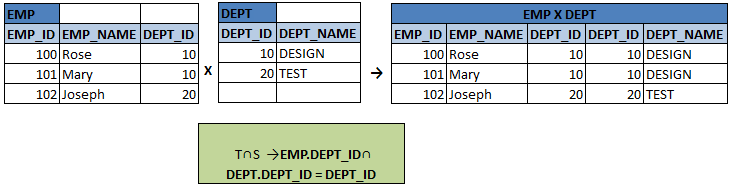• T∩S = {A}, which is some set of records from both T and S, then size estimate of join between T and S is nT * nS / dAS. i.e.; it is equivalent to total number of cross joins divided by distinct values in table S. Suppose DESIGN_EMP and TEST_EMP has 10 and 6 records respectively. Suppose DESIGN_EMP has 6 distinct values and TEST_EMP has 5 distinct values. Suppose they have 3 records in common. Then size estimate of join is given with DESIGN_EMP as 10*6/6 = 10 and with TEST_EMP as 10*6/ 5 = 12. Since the size estimate with DESIGN_EMP is less, it is used as the accurate estimate.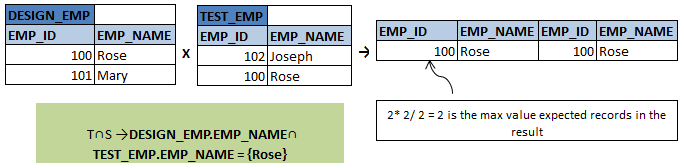Outer Joins

In the case of outer joins, we have few extra records than a normal joins. Hence the size estimate of outer joins is calculated as sum of size estimate of normal joins between two tables and the size of one table, say T. Suppose we have left outer join between tables T and S: T ∞ LEFT OUTER JOINS, then size estimate of it is sum of size estimate of T ∞ S and size of T. In the case of right outer join, it is the sum of size estimate of T ∞ S and size of S. If it is a full outer join then size estimate of it is sum of size estimate of T ∞ S size of T and size of S. Here while calculating size estimate of normal join, 5 different cases explained above are considered and estimated accordingly.

Data Independence

For example, assume left outer join between EMP and PROJECT. Let EMP has 1000 records and PROJECT has 30 records. The left outer join between these two tables will have normal join between them for the matching records and all other records from EMP for which there is no match in PROJECT. Hence its size estimate is given as (1000 * 30) + 1000 = 31000(assuming T∩S = ?).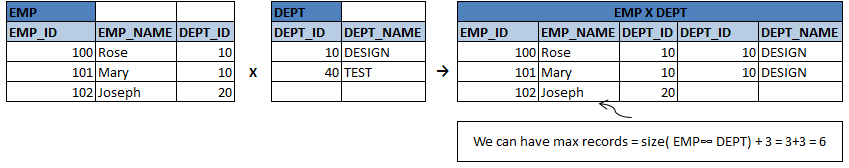In the case of right outer join, above size estimate would be (1000 * 30) + 30 = 30030 and in the case of full outer join, it is (1000 * 30) + 1000 + 30= 31030.

Projection

This is the operation of selecting particular column/s from a table. Hence its size estimate is equal to number of distinct values of column/s present in the table.
∏A (T) = d AT
Size estimate of selecting the column EMP_ID in EMP table is equal to total number of records in EMP, since EMP_ID is the primary key. Let us assume there are only 6 different values for AGE in EMP. Then size estimate for projecting AGE from EMP is 6.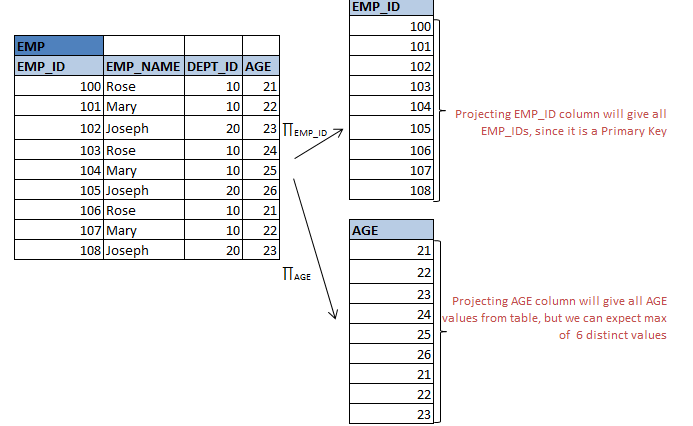Aggregation

This is similar to projection. Here distinct values of column are grouped and their aggregate values (like count, sum, max, min etc) are calculated. Hence its size estimate is equal to number of distinct values of column present in the table.

Size estimate for calculating number of employees in different department is equal to total number of distinct department present in the table.

Set Operation

Suppose we have set operation UNION or INTERSECTION on the same table T. Then it is same as size estimate for selection operation. i.e.; when we have union operation on same table with two different select condition, then it is same as applying select condition in sequence or by using AND operator.

Characteristics of a Database

σ θ1 (T) Uσθ2 (T) =σθ1σθ2 (T)

σ θ1 (T) ∩σθ2 (T) =σθ1σθ2 (T)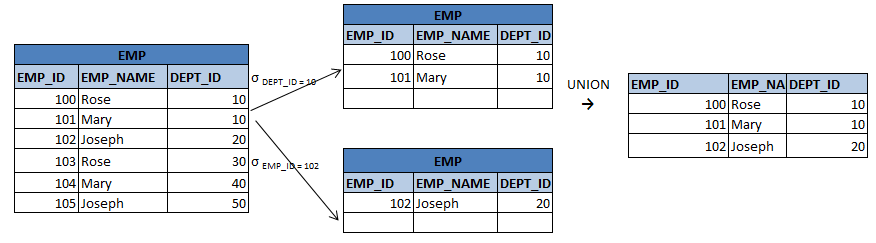Suppose we have set operation UNION or INTERSECTION on the different tables T and S. Then

Size estimate for T U S: size of T + size of S
Size estimate for T U S: min (size of T, size of S)
Size estimate for T - S: size of T

i.e. when union operation is used, records of both the tables are combined. Hence it is the sum of size of both tables. When intersection operation is used, it will have only the matching records of both tables. Hence the result set will have utmost size of T or S records, whichever is smaller. When we do difference operation, we can have utmost records from first table or less. All these estimates of set operations are the maximum values of size the result set can have. But in most of the cases, it will be lesser than the estimated value.

Distinct Values

Let us see what happens to size estimate when we are selecting or joining the tables for distinct values.

• Selection : Suppose we have a selection operation with theta join, σ θ (T). Suppose theta join is defined on column A. Then theta forces to take specified value of A (equal operator), then size estimate is equal to one. i.e.; it will return at least one record with matching the condition. When theta forces to take some specified values from the set of values of A,  then  the size estimate is equal to distinct values of A i.e.; d AT.

Suppose we have σ AGE = 18 (EMP), then it is assumed that at least one record with AGE = 18 would be pulled. There is a chance that no records present with this condition too. When we have condition like σ AGE <=21 (EMP), we select a group of values from EMP with different ages. Hence there could be distinct values of AGE <=21 records could be returned i.e.; assume in EMP min value of age is 18. Then at least 4 records with age 18, 19, 20 and 21 would be retrieved, but necessary that there exists at least one record for each age. There may or may not be records with those ages. These are all assumptions.

Structure of DBMS

In general the size estimate of theta selection would be min (dAT, n σθ (T) i.e.; the number of records selected would completely depend on either distinct values of column value or number of records in selection.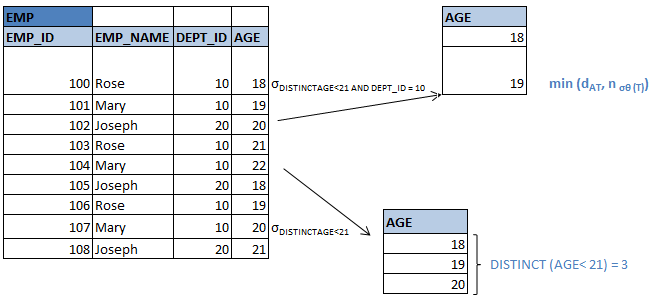• Joins : Suppose we have join operation between tables T and S. Suppose A is the column from T. then size estimate is given as min (dAT, n T∞S) i.e.; Suppose we have join between EMP and DEPT to find out the manager details of the department. Let department can have only one manager. Then join between these two tables will have records same as distinct values of department, d AT.

Suppose we have join to find out the employee details of particular department. Then size estimate is not equal to distinct values of department, but the actual join between them.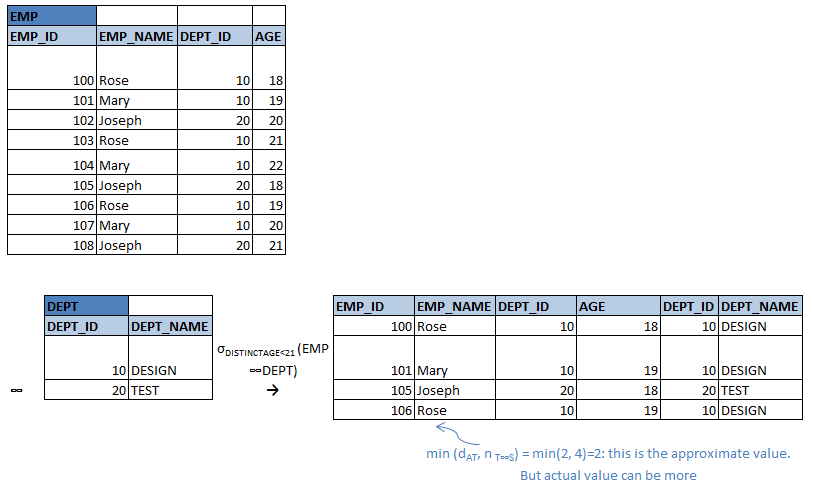Suppose we have A1 from table T and A2 from table S, the size estimate for table T be V (T), for table S be V(s), for table T without A2 values be V(A1-A2), and for table S without A1 be V(A2-A1). Then the join between them is given by min (V (T)* min (V(A2-A1), V(S), V(S)*min (V(A1-A2)*V(T)), n T ∞S)

• Distinct and Aggregation : The size estimate for finding distinct and aggregation are same as number of distinct values of column, dAT; same as projection or aggregation as we saw above.